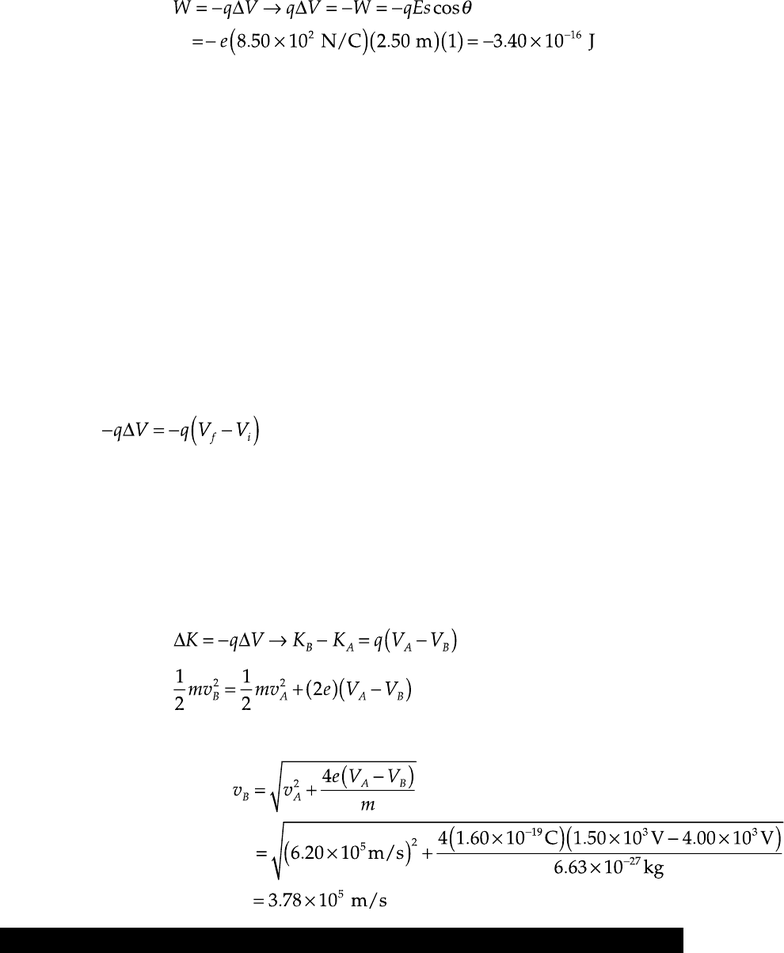Class Notes (1,100,000)
CA (640,000)
UTSG (50,000)
BIO (2,000)
BIO120H1 (1,000)
Lecture

# BIO120H1 Lecture Notes - Electric Potential Energy, Electric Field, Robert Andrews Millikan

Department
Biology
Course Code
BIO120H1
Professor
Jennifer Harris

This preview shows pages 1-3. to view the full 19 pages of the document.25
Electric Potential
CHAPTER OUTLINE
25.1 Electric Potential and Potential Difference
25.2 Potential Difference in a Uniform Electric Field
25.3 Electric Potential and Potential Energy Due to Point Charges
25.4 Obtaining the Value of the Electric Field
from the Electric Potential
25.5 Electric Potential Due to Continuous Charge Distributions
25.6 Electric Potential Due to a Charged Conductor
25.7 The Millikan Oil-Drop Experiment
25.8 Applications of Electrostatics
* An asterisk indicates a question or problem new to this edition.
OQ25.1 Answer (b). Taken without reference to any other point, the potential could have
any value.
OQ25.2 Answer (d). The potential is decreasing toward the bottom of the page, so the
electric field is downward.
OQ25.3 (i) Answer (c). The two spheres come to the same potential, so Q/R is the same
for both. If charge q moves from A to B, we find the charge on B:
Sphere A has charge 450 nC 300 nC = 150 nC.
(ii) Answer (a). Contact between conductors allows all charge to flow to the
exterior surface of sphere B.

Only pages 1-3 are available for preview. Some parts have been intentionally blurred.OQ25.5 Ranking a > b = d > c. The potential energy of a system of two charges is
The potential energies are: (a) (b) (c)
(d)
OQ25.6 (i) Answer (a). The particle feels an electric force in the negative x direction. An
outside agent pushes it uphill against this force, increasing the potential
energy.
(ii) Answer (c). The potential decreases in the direction of the electric field.
OQ25.7 Ranking D > C > B > A. Let L be length of a side of the square. The potentials are:
OQ25.8 Answer (a). The change in kinetic energy is the negative of the change in electric
potential energy:
OQ25.9 Ranking c > a > d > b. We add the electric potential energies of all possible pairs.
They are:
(a)
(b)
(c)
(d)
OQ25.10 Answer (b). All charges are the same distance from the center. The potentials
from the +1.50-µC, 1.00-µC, and 0.500-µC charges cancel.

Only pages 1-3 are available for preview. Some parts have been intentionally blurred.OQ25.11 Answer (b). The work done on the proton equals the negative of the change in
electric potential energy:
OQ25.12 (i) Answer (b). At points off the x axis the electric field has a nonzero y
component. At points on the negative x axis the field is to the right and
positive. At points to the right of x = 0.500 m the field is to the left and
nonzero. The field is zero at one point between x = 0.250 m and x = 0.500 m.
(ii) Answer (c). The electric potential is negative at this and at all points because
both charges are negative.
(iii) Answer (d). The potential cannot be zero at a finite distance because both
charges are negative.
OQ25.13 Answer (b). The same charges at the same distance away create the same
contribution to the total potential.
OQ25.14 The ranking is e > d > a = c > b. The change in kinetic energy is the negative of the
change in electric potential energy, so we work out
in each case.
(a) (e)(60 V 40 V) = +20 eV (b) (e)(20 V 40 V) = 20 eV
(c) (e)(20 V 40 V) = +20 eV (d) (e)(10 V 40 V) = +30 eV
(e) (2e)(60 V 40 V) = +40 eV
OQ25.15 Answer (b). The change in kinetic energy is the negative of the change in electric
potential energy:
Solving for the speed gives